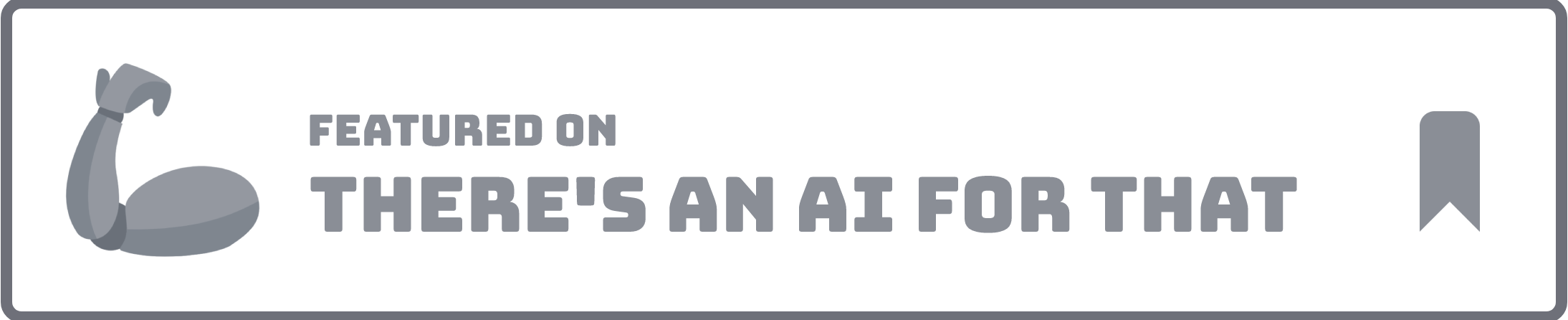Excel formulas 2023-07-23# Excel Formul Bot

No ratings
Overview
Generated by ChatGPT

The Excel Formula Bot is a website that utilizes natural language processing algorithms to generate Excel or Google Sheet formulas based on simple text prompts.

It aims to enhance the efficiency of writing formulas by providing AI assistance.One of the key features of the Excel Formula Bot is its ability to seamlessly generate Excel or Google Sheets formulas using straightforward text-based prompts.

This eliminates the confusion often associated with complex formulas and allows users to easily handle them with AI assistance.Additionally, the Excel Formula Bot can generate VBA code through user-friendly text prompts, requiring minimal effort from the user.The website offers a free plan with 5 credits, allowing users to test the functionality and quality of the service before considering a paid plan.While the Excel Formula Bot strives to provide accurate formulas, it is recommended that users review and verify the generated formulas to ensure they meet their specific needs.The tool is capable of handling a wide range of formula complexities, including nested functions, multiple conditions, and advanced calculations.

However, extremely complex or specialized formulas may require manual intervention or additional customization.Accepted payment methods for the paid plans include credit cards, debit cards, and PayPal.Overall, the Excel Formula Bot streamlines the process of generating Excel or Google Sheet formulas by leveraging AI-powered natural language processing, providing users with a convenient and efficient solution.

Excel Formul Bot was manually vetted by our editorial team and was first featured on July 23rd 2023.Promote this AI Claim this AI

## Would you recommend Excel Formul Bot?

Help other people by letting them know if this AI was useful.

Inside the forest, an 18-year-old human boy is riding on the back of a big brown wolf, and when you press the w button on the keyboard, both the brown wolf and the human boy riding on the back of the wolf turn into white smoke for a few seconds, and then return to their original state.

## 20 alternatives to Excel Formul Bot for Excel formulas

•Generated and explained Excel/Sheets formulas.
1,318
1
5.0
•Creating complex Excel formulas and macros.
113
•82
•Excel formulas generated with assistance.
72
1.0
•47
•Instant Excel formula generation from text instructions.
45
•Simplified complex calculations in Excel.
44
1
5.0
•Simplified creation and understanding of Excel formulas.
35
•Analyzed data, collaborated, improved productivity.
31
•Excel formula suite for smarter productivity.
29
•25
•Streamlined Excel formula generation from instructions.
25
3
3.0
•Automates Excel formula creation with explanations.
21
•Summarizes and categorizes text in spreadsheets.
14
•Improved productivity through automating Excel/Sheets.
14
•Formula & Query Gen. for Spreadsheets & Databases.
10
•Excel formulas
8
•Excel productivity with efficient formula generation.
8
•Excel Bot simplifies formulas & generates VBA code
7
•Data analysis, formulas, and preparation streamlined.
6

## Pros and Cons

### Pros

Generates Excel formulas
Uses text prompts
Efficiency enhancer
Generates VBA code
Free plan available
Supports complex formulas
Nested functions capability
Multiple condition handling
Variety of payment methods
Accuracy in formula generation
Requires minimal user effort
Ease of use
Review and verify functionality
Streamlines formula generation process
Handles wide range of complexities
Handles both Excel and Google Sheets
Prompt service

### Cons

Limited free plan
May require manual interventions
Does not guarantee accuracy
May struggle with specialized formulas
No API mentioned
Cannot handle extreme complexities
Does not support non-credit payments
No offline mode mentioned

## Q&A

What is Excel Formula Bot?
How does the Excel Formula Bot work?
What can I get with Excel Formula Bot's free plan?
What types of formulas can the Excel Formula Bot generate?
Why is it important to review and verify the formulas generated by the Excel Formula Bot?
Can Excel Formula Bot handle advanced calculations and nested functions?
What are the limitations of Excel Formula Bot in generating formulas?
What payment methods are accepted by Excel Formula Bot?
Does Excel Formula Bot offer a paid plan?
Can Excel Formula Bot generate formulas for Google Sheets as well as Excel?
How does Excel Formula Bot use AI to generate formulas?
What kind of text prompts does Excel Formula Bot accept?
Can Excel Formula Bot generate VBA code?
How efficient is Excel Formula Bot?
Do I need to understand complex Excel formulas to use Excel Formula Bot?
What distinguishes the Excel Formula Bot from other formula generating tools?
Why should I use Excel Formula Bot instead of manually writing my formulas?
Can Excel Formula Bot help me understand complex formulas?
Can Excel Formula Bot handle multiple conditions in a formula?
What do I do if the generated formula does not meet my specific needs?

## If you liked Excel Formul Bot

•1,318
1
5.0
•51
5.0
•4
•3
•2
•23
••3
•70
•12
•55
1
2.0
•36
3
5.0

## People also searched

### Help

+ D bookmark this site for future reference
+ ↑/↓ go to top/bottom
+ ←/→ sort chronologically/alphabetically
Enter open selected entry in new tab
⇧ + Enter open selected entry in new tab
⇧ + ↑/↓ expand/collapse list
/ focus search
Esc remove focus from search
A-Z go to letter (when A-Z sorting is enabled)
+ submit an entry